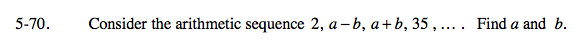### Home > A2C > Chapter 5 > Lesson 5.2.1 > Problem5-70

5-70.Since this sequence is arithmetic, what has to be true about the differences between terms?

How many times would you add the difference to get from 2 to 35?

2 + d + d + d = 35

d = 11

Since you know the first term and the common difference, you can write all four terms of the sequence.

Use the sequence to write a system of equations: ab = ? and a + b = ?

a = 18.5, b = 5.5